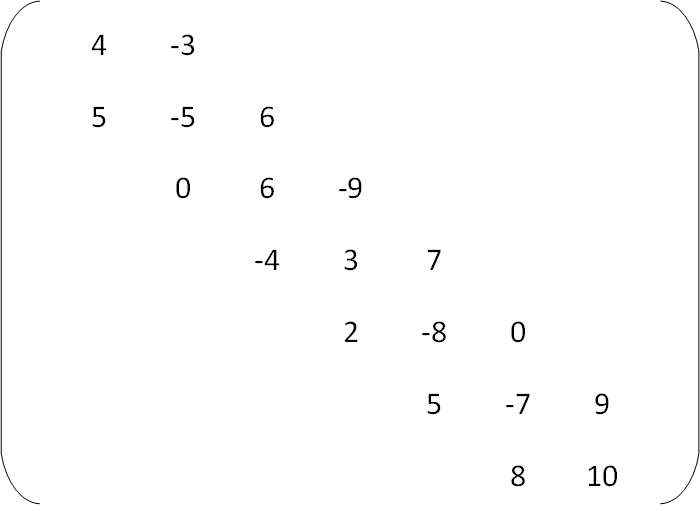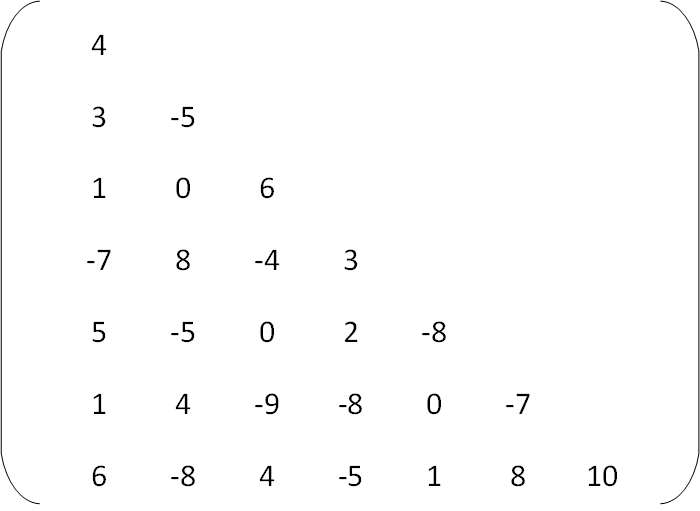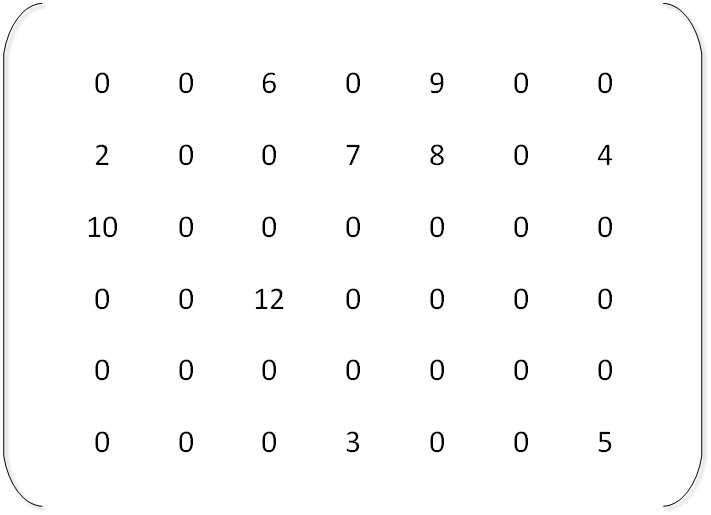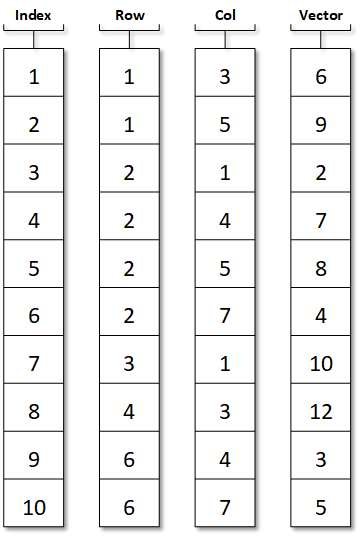# Array C Programing

Sparse Matrix

A matrix is a two-dimensional object with a total of m x n values made up of m rows and n columns. If most of the matrix elements are worth 0, then Its is called sparse matrix.

or,

Matrices with a relatively high proportion of zero entries are called sparse matrices. Two general types of n-square sparse matrices, which occur in various applications, are:

• Tridiagonal Matrix
• Triangular Matrix

The  triangular matrix, where all entries where above the main diagonal are zero equivalently where zero entries can only occur on or below the main diagonal is called a lower Triangular Matrix.The matrix, where non-zero entries can only occur on the diagonal or on elements immediately above or below the diagonal is called a Tridiagonal matrix.The natural method of representing matrices in memory as 2-D arrays may not be suitable for sparse matrices. That is, one may save space by storing
only those entries, which may be non-zero. This can be explained with the following example:Non-zero element are:

M = 6, M = 9,

M = 2, M = 7,

M = 8, M = 4,

M = 10, M = 12,

M = 3,M = 5;

One of the basic methods for storing such, a sparse matrix is to store non-zero elements in a one-dimensional array and to identify each array element with row and column indices as:The ith element of vector V is the matrix element with row; column indices Row[i] and Col[i].